## Matrix Sum

To compute the matrix sum, we need to specify the direction of the sum.

The default matrix sum direction is the total sum, which adds all elements in the matrix to produce a single number. Mathematically it is symbolized by double sums.

When matrix sum direction is row, we sum all rows in each column to produce a row vector .

When matrix sum direction is column, we sum all columns in each row to produce a column vector .

Example: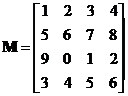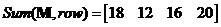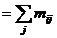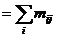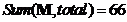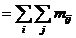The interactive program below is designed to help you to compute matrix sum in any of the above direction. Random Example will generate random matrix at random size.

Matrix direction